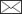Pekka Kilkki, Martti Varmola

A nonlinear simultaneous equation model to determine taper curve.

Kilkki P., Varmola M. (1979). A nonlinear simultaneous equation model to determine taper curve. Silva Fennica vol. 13 no. 4 article id 5047. https://doi.org/10.14214/sf.a14904

Abstract

In the original set of equations derived by regression analysis, relative-height diameters (endogenous variables) are presented as nonlinear functions of the other relative-height diameters and of the height of the tree (an exogenous variable). Any of the original equations can be replaced by an interpolation formula which links a measured diameter to the four closest relative-height diameters. The solution of the simultaneous equation model yields 10 relative-height diameters. Intermediate values are obtained to avoid biases due to the nonlinearity of the simultaneous model equations.

The PDF includes a summary in Finnish.

Published in 1979

Views 921

Available at https://doi.org/10.14214/sf.a14904 |Register
Click this link to register to Silva Fennica.